# 4.3.5. Boosting¶

Given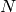training data points and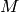classifiers. The AdaBoost algorithm does the following:

1. Initialize weights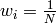2. For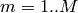:

1. Fit the classifier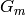to the training data using weights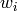2. Compute the classifier error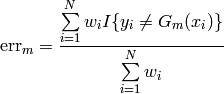1. Compute the classifier weight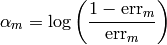1. Recompute all data weights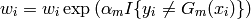3. The combined classifier is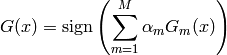## 4.3.5.2. Interfaces¶

Bases: ailib.fitting.model.Model

Given M (weak) classifiers, AdaBoost iteratively trains each one of them with respect to so far misclassified samples. For a formal representation, consult the respective documentation.

As can be seen from the schematics, the classifiers are required to support weighted samples. The classifiers have to be linked to the instance of this class before training. This can be achieved through calls to the AdaBoost.addModel() method.

Add a trainable model to the set of boosted classifiers.

The models are required to support weighted samples.

Parameter: m (Model) – Model
err((x, y))
Return the distance between the target y and the model prediction at the point x.
eval(x)

Evaluate the model at data point x.

The outcome is the majority vote over the outcome of all classifiers w.r.t. their weight (note the formal representation).

fit(data)

Apply the AdaBoost algorithm on the presented data set.

Parameter: data – Training data. self